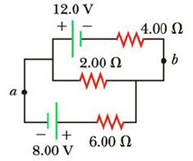Chapter 18, Problem 20P

Chapter
Section
Textbook Problem

For the circuit shown in Figure P18.20, calculate (a) the current in the 2.00-Ω resistor and (b) the potential difference between points a and b, ΔV= Vb− Va.Figure P18.20

(a)

To determine
The current in 2.00Ω resistor.

Explanation

Given Info:

Resistance R1 is 4.00Ω , Resistance R2 is 2.00Ω , Resistance R3 is 6.00Ω , Voltage ε1 is 12.0V , and Voltage ε2 is 8.00V .

Explanation:

Below Figure shows the direction of current in the circuit

• ε1,ε2 is voltage in the circuits
• R1,R2,R3 is corresponding resistance in the circuits
• I1,I2,I3 is corresponding current in the circuits

Apply Kirchhoff’s voltage law to the left and right loop.

From the upper loop

ε1R1I1R2I2=0

Substitute, 4.00Ω for R1 , 2.00Ω for R2 , and 12.0V for ε1 in the above equation to find expression for I1

12.0V4.00ΩI12.00ΩI2=012.0V(2.00Ω)I2=4.00ΩI13.00V(0.500Ω)I2=I1

From the lower loop

ε2+R2I2R3I3=0

Substitute, 2.00Ω for R2 , 6

(b)

To determine
The potential difference between point a and b.

Still sussing out bartleby?

Check out a sample textbook solution.

See a sample solution

The Solution to Your Study Problems

Bartleby provides explanations to thousands of textbook problems written by our experts, many with advanced degrees!

Get Started

The best way to control salt intake is to cut down on processed and fast foods. T F

Nutrition: Concepts and Controversies - Standalone book (MindTap Course List)

Complete the following table:

Chemistry & Chemical Reactivity

5-50 How many molecules of CO are in 100. L of CO at STP?

Introduction to General, Organic and Biochemistry

An __________ is a relationship in which one organism lives inside another.

Biology: The Unity and Diversity of Life (MindTap Course List)